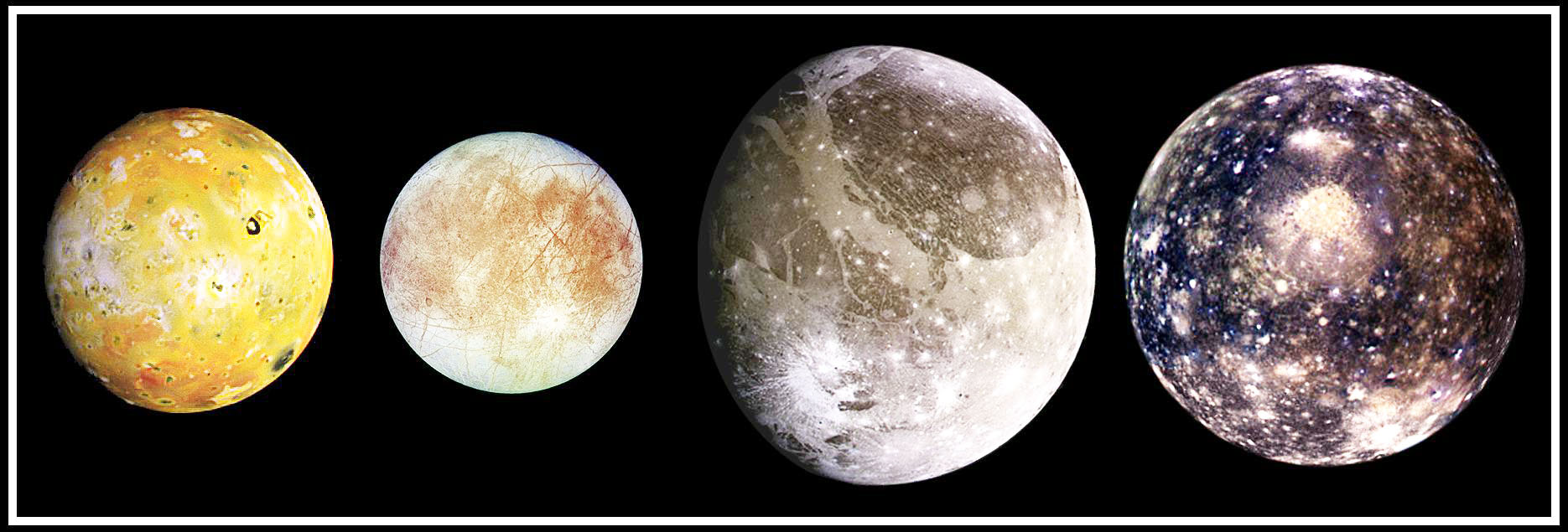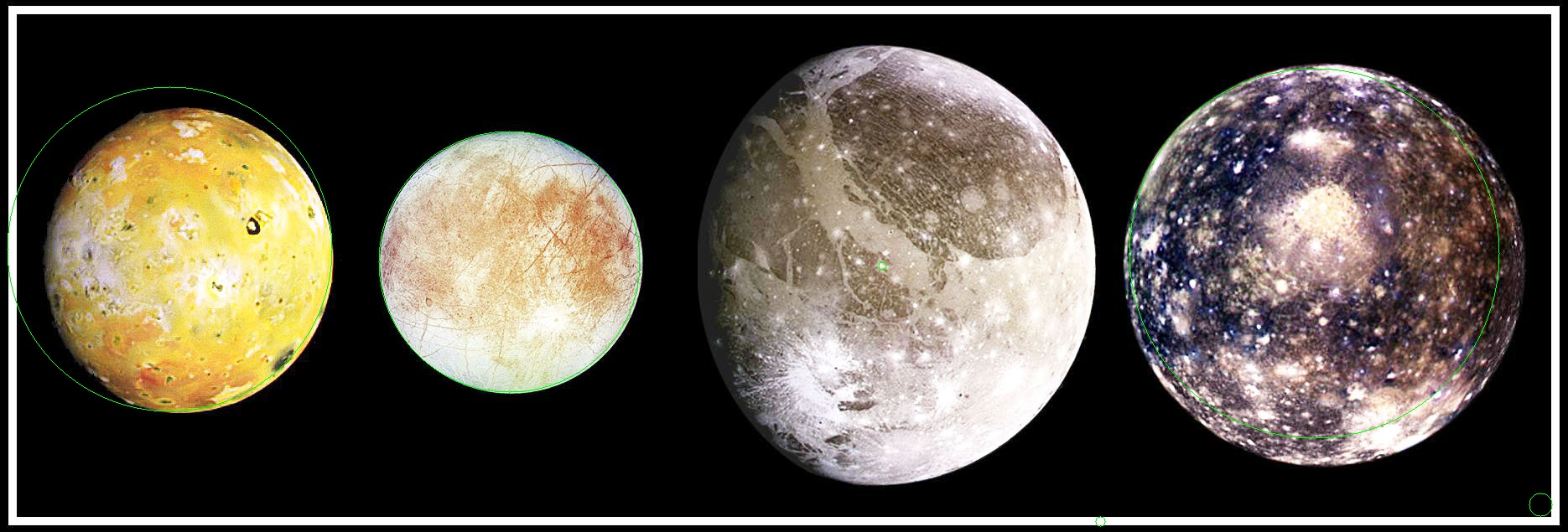# Sep 13 2017 Detecting circles in an image with Hough transforms

Part of a series of examples from the CV cheat sheet. Click here for other computer vision applications.

Detecting circles in an image is a common task in computer vision. One way to do this is with the Hough (pronounced "Huff") transform. The math behind it is beyond the scope of this example, but we should be able to get you off the ground using it with OpenCV and Python. We're going to assume you have OpenCV installed already and are able to use it in Python.

Take an input image, say, these solar system moons:Let's detect the circles in this image. The gist is this:

1. Open an image
2. Detect circles in it
3. Draw those circles back on the image (you don't need to do this necessarily, but it's a nice visual)

Here's the code to extract circles in Python:

 ``` 1 2 3 4 5 6 7 8 9 10 11 12 13 14 15 16 17 18 19 20 21 22 23 24 25 26``` ``````# import libraries we'll need import cv2 import numpy as np # read in your image img = cv2.imread('moons.jpg') # convert to grayscale gray = cv2.cvtColor(img, cv2.COLOR_BGR2GRAY) # detect circles in the image # dp and minDist are parameters that you can tune depending on # your data. # dp is the accumulator resolution. Think of it as accuracy of circle center location. Lower values take longer # minDist is the minimum distance permitted between circle centers # param1 is the upper threshold for the edge detection preprocessing. If you have low-contrast circles, increasing this may help # param2 is # param1 and param2 are optional. circles = cv2.HoughCircles(img, cv2.HOUGH_GRADIENT, dp=5, minDist=400, param1=350, param2=25) # circles are returned in (x, y, radius) format for x, y, radius in circles: cv2.circle(img, (x, y), radius, (0, 255, 0), cv2.LINE_AA) # write the image to disk cv2.imwrite('houghcircles.jpg', img) ``````

Here's the result:As you can see, there is still some error! The most elliptical of our Galilean satellites wasn't detected at all, and the radius of a couple others is off. Tweaking the parameters for your use case will help this. If you want to count almost-circles or not count them, how sharp the edge needs to be to count as a detection, and so on.